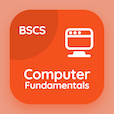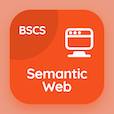Online CS Degree Courses

Digital Logic Design MCQs

Digital Logic Design MCQ - Topics

Practice Introduction to Combinational Logics Multiple Choice Questions (MCQ Quiz), Introduction to Combinational Logics quiz answers PDF to study digital logic design course for digital logic design online classes. Combinational Logics Multiple Choice Questions and Answers (MCQs), Introduction to Combinational Logics quiz questions for top computer science schools. Introduction to Combinational Logics Book PDF: introduction to combinational logics, subtractors in combinational logics, half adders, design procedure in combinational logics test prep for associates in computer science.

"Circuits that employ memory elements in addition to gates is called" MCQ PDF: introduction to combinational logics App APK with combinational circuit, sequential circuit, combinational sequence, and series choices for top computer science schools. Learn introduction to combinational logics quiz questions for merit scholarship test and certificate programs for online degrees.

## MCQ on Introduction to Combinational Logics Quiz

MCQ: Circuits that employ memory elements in addition to gates is called

combinational circuit
sequential circuit
combinational sequence
series

MCQ: Logic gates takes input signals and generates signals to

within gate
input
output
circuit

MCQ: Variable in Boolean expressions can be expressed as

primed
unprimed
even
both a and b

MCQ: Circuits whose output depends on directly present input is called

combinational circuit
sequential circuit
combinational sequence
series

MCQ: An integrated circuit with internal logic gates connected through electronic fuses is called

portable large device
portable logic device
programmable large device
programmable logic device

### More Quizzes on Digital Logic Design Book